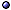Representations in Quantum Theory

In General > s.a. wigner functions [phase-space representations].
* Idea: The basic problem in the quantum theory of a physical system is choosing a complete set of observables that characterize the states one wants to describe, and find a representation of this set on a Hilbert space.
* Issues: How unique is the representation? Which functions on the basic operators can/should one represent?
* Relevant tools / results: The Stone-von Neumann theorem, Van Hove theorem, GNS construction.
@ General references: Shewell AJP(59)jan [operator ambiguities]; de la Torre AJP(02)mar-qp/02 [including aX + (1−a)P, $$1\over2$$(XP + PX)]; Halvorson SHPMP(04)qp/01 [and complementarity]; Vourdas JPA(06) [analytic, rev]; Bracci & Picasso AJP(07)mar [inequivalent, Aharonov-Bohm effect example]; Blood a1310 [what kets represent]; Stepanian & Kohandel a1312 [unitarily inequivalent representations].
@ Related topics: Fuss & Filinkov a1406 [periodic quantum systems, Colombeau algebra of generalized functions]; > s.a. Superseparability; Weyl Algebra.Main types: see configuration-space based representations [Schrödinger, Heisenberg, interaction pictures].

Bargmann-Segal (Coherent State) Representation > s.a. coherent states [including Segal-Bargmann transform]; deformation quantization.
* Idea: The holomorphic representation on L2($$\mathbb C$$, exp{− |z|2} dz dz*) (ψ(z) is analytic), obtained from the usual L2($$\mathbb R$$,dx) using the heat kernel ρt by the transformation

ψ(x) $$\mapsto$$ (Ct ψ)(z):= dx ρt(zx) ψ(x) .

* Inner product, operators: If ψ(z):= ∑n=0 (n!)−1/2 zn $$\langle$$n|ψ$$\rangle$$, with |a$$\rangle$$ an eigenvector of a, then

$$\langle$$ψ|φ$$\rangle$$:= π−1 ψ*(z) φ(z) exp{−z*z} d2z ;
a ψ(z) = z ψ(z)   and   a ψ(z) = (∂/∂z) ψ(z) .

* Generalizations: The transform can be generalized to functions on groups.
* For the simple harmonic oscillator: Define the complex variable as z:= (ωm/2$$\hbar$$)1/2 q + i (2$$\hbar$$ωm)−1/2 p.
@ General references: Bargmann CPAM(61), PNAS(62); Hall CM-qp/99, CMP(02) [compact groups, geometric quantization]; Villegas-Blas JMP(02) [kernel of transform]; Hübschmann JGP(08)m.DG/06 [and holomorphic Peter-Weyl theorem]; Vourdas et al JPA(12)-a1111 [generalized]; Oeckl JMP(12)-a1109 [isomorphism with the Schrödinger representation, field theory]; Bergeron et al PLA(13) [equivalence to Weyl quantization].
@ Related representations: Ribeiro et al PRL(05), Ribeiro et al JPA(09)-a0809 [conjugate representation]; Parisio PTP(10)-a1003 [off-center coherent-state representation]; Viscondi et al a1510 [generalized coherent-state representation, semiclassical propagator].
@ Other systems: Kowalski & Rembieliński JMP(01)qp/00 [particle on S2]; Aldaya & Guerrero JPA(93) [relativistic oscillator]; Ashtekar et al JFA(96)gq/94 [spaces of connections]; Villegas-Blas JMP(06) [for L2(Sn)]; > s.a. quantum oscillators; wigner functions.

Other Types of Representations > s.a. fock space; momentum representation; non-commutative physics; Polymer Representation.
* Tomography: A formulation of quantum mechanics without probability amplitudes, expressed entirely in terms of observable probabilities; In it quantum states are represented not by complex state vectors or density matrices, but by real "probability tables" or marginal distribution functions, whose time dependence is governed by a classical evolution equation.
@ Probability representation, tomography: Wootters FP(86); Man'ko et al PRA(98)qp [Green's functions], JPA(03) [identical particles], PLB(98)ht [in quantum field theory], PLA(06), qp/06-conf [in abstract Hilbert spaces], RPMP(08)qp/06; Weigert PRL(00)qp/99, qp/99 ["expectation-value representation" for spins, similar?]; Howard & March PLA(06) [and momentum density]; Caponigro et al FdP(06)qp; Man'ko et al qp/06 [bibliography]; Kiukas et al PRA(09)-a0902; Ibort et al PS(09)-a0904 [intro]; Andreev et al JRLR-a0910 [for fermion fields]; Fuchs PiC-a1003, a1003 [quantum Bayesian viewpoint]; Ibort et al PLA(10)-a1004; Man'ko & Man'ko AIP(11)-a1102 [dynamical symmetries and entropic uncertainty relations]; Korennoy & Man'ko a1104 [propagator]; Man'ko & Ventriglia IJGMP(12)-a1111-conf [free particle motion, classical and quantum]; Ibort et al PS(11)-a1204 [C*-algebraic approach]; Man'ko & Man'ko AIP(12)-a1208 [tomographic entropic uncertainty relations]; Fedorov PLA(13) [Feynman integral and perturbation theory]; Aniello JPCS(13)-a1310 [evolution and semigroups]; Man'ko & Man'ko EPJWC(14)-a1403 [and Wigner functions]; Korennoy & Manko a1511 [gauge transformation of states]; López-Yela a1512-PhD; Korennoy & Man'ko IJTP(17)-a1610 [symplectic and optical joint probability distributions]; Man'ko et al a1905; > s.a. entropy; quantum states [reconstruction]; in quantum field theory and in quantum gravity; wigner functions.
@ Weil representation: Gurevich & Hadani a0808 [in characteristic two].
@ Weyl representation: Parthasarathy a1803 [and Lévy processes].
@ Related topics: Floyd qp/03-proc [trajectory representation, high-energy limit]; Torres-Vega PRA(07) [energy-time]; Chmielowiec & Kijowski JGP(12)-a1002 [generalized, fractional Fourier transform]; Aerts & Sassoli de Bianchi JMP(16)-a1504 [extended Bloch representation, interference and entanglement]; Chabaud et al PRL(20)-a1907 [stellar representation]; > s.a. formalism [operator ordering]; Stone-von Neumann Theorem; tests of quantum mechanics.